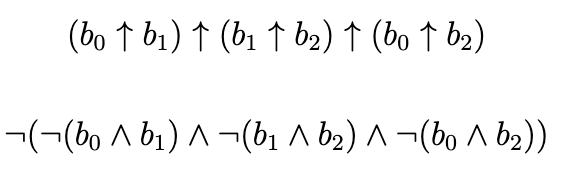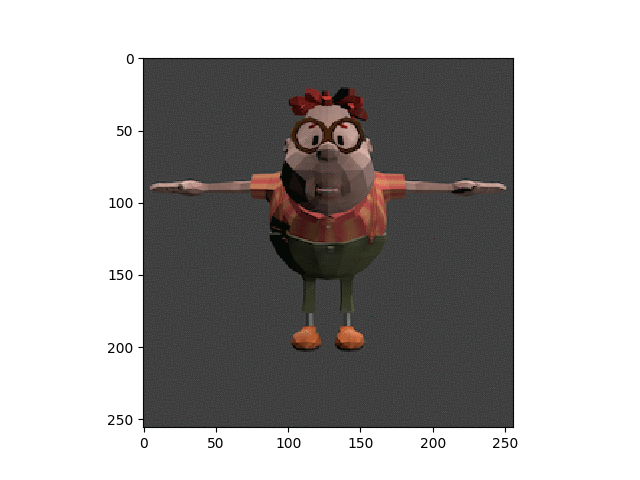## Simple Triple Modular Redundancy

I recently went back to UGA Hacks to give a workshop / talk on Triple Modular Redundancy(TMR). I wanted to have a very visual tool to help illustrate the concept of TMR, so I made a simple python program with a GUI. The program shows an image slowing experiencing bitflips until the original image is indistinguishable. This is, of course, hyperbole and bitflips do not occur this quikly - I found it to be a useful teaching tool.

Fundamentally, TMR is exactly what it says it is. There are 3 copies of something and you can check values at particular locations to make sure all copies are still equal. If you notice that the copies are not equal, i.e. one copy is 0 and the other two are 1, then you can choose to correct the copy that is the outlier. As a logic circuite this looks like:And logically, assuming your bits are b0, b1, and b2:In python this could be done with:

if (not((not (b and b1)) and (not (b1 and b2)) and (not (b0 and b2)))):
# do some TMR here


All of this code is on github here: https://github.com/piepieninja/simpleTMRexample

In the TMR.py file, located in the root of the github repo, there is an example setup to test some TMR algorithms. In this example an image of carl has 1 bitflip occuring every millisecond. This is a large exaggeration, only to demonstrate the concept of TMR. These bitflips can be seen below:to attempt to slow the degridation of the image, TMR can be performed. In these examples the TMR algorthm occurs at a slower rate than bitflips occur. This is done to reflect the reality TMR is only capible of slowing the onslaught of radiation.